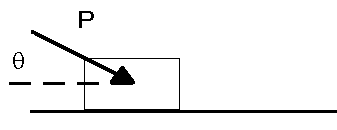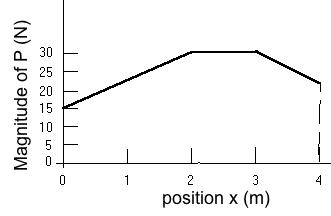Teaching Physics with the Physics Suite Edward F. Redish Problems Sorted by Type | Problems Sorted by Subject | Problems Sorted by Chapter in UP

Work-energy, impulse-momentum, and N2*

We have developed the concept of energy to allow us to express the results of Newton's laws in different ways. In this problem, you will calculate the motions using energy and will compare it to calculating the motion using Newton's second law (and the definitions of average velocity and acceleration).

A block with mass 12.0 kg is being pushed along a horizontal floor by a force P as shown in the following diagram. At clock reading t=0.00 s and position x = 0.00 m the block has an instantaneous velocity of 2.00 m/s. The angle is θ = 0.35 rad. The force P is applied to the block at the instant it is in position x = 0.00 m. The direction of P remains fixed, but its magnitude varies with position as shown in the graph at the right. The coefficient of sliding friction between the block and the floor is 0.2.1. Calculate the velocity the box has at the position x = 4.00 m by calculating the net work done on the block during the diplacement and finding the final kinetic energy.
2. Try doing the calculation of the final velocity using Newton's second law and the definitions of velocity and acceleration. If you get stuck, explain you difficulty in completing the calculation.
3. Calculate and describe any internal thermal energy changes of the block-floor system over the entire history of the motion dealt with above.
4. Calculate the momentum of the block at position x = 4.00 m, the momentum change imparted to the block over the interval between positions x = 0.00 and x = 4.00 m, and the net impulse that must have been delivered to the block over this interval.

* Based on a problem in [Arons 1990].